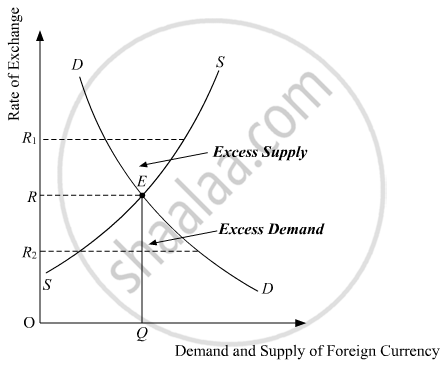Share
Notifications

View all notifications
Books Shortlist
Your shortlist is empty

# How is exchange rate determined in the foreign exchange market? Explain. - CBSE (Arts) Class 12 - Economics

Login
Create free account

Forgot password?
ConceptDetermination of Exchange Rate in a Free Market

#### Question

How is exchange rate determined in the foreign exchange market? Explain.

#### Solution

In the foreign exchange market, the equilibrium exchange rate is determined by the intersection of the demand curve for foreign currency and the supply curve of the foreign currency.In the above diagram, DD is the demand curve for foreign currency and SS is the supply curve of foreign currency. The point E is the point of intersection, where the demand curve and the supply curve intersects. Thus, the point E represents the equilibrium exchange rate. OR is the equilibrium exchange rate and OQ  is the quantity demanded and supplied of the foreign currencies.

If exchange rate rises to OR1, then the supply of foreign currency exceeds the demand for foreign. This will then force the exchange rate to fall back to OR due to the excess supply. On the contrary, if the exchange rate falls to OR2, then there exists excess demand. Consequently, the rate of exchange rises from OR2 to OR.

Thus, in the foreign exchange market, the exchange rate is determined by the intersection of the demand and supply of the foreign exchange. In case of any mismatches between the demand and the supply, then such mismatches are corrected automatically by the invisible hands of the market (demand and supply).

Is there an error in this question or solution?

#### APPEARS IN

Solution How is exchange rate determined in the foreign exchange market? Explain. Concept: Determination of Exchange Rate in a Free Market.
S